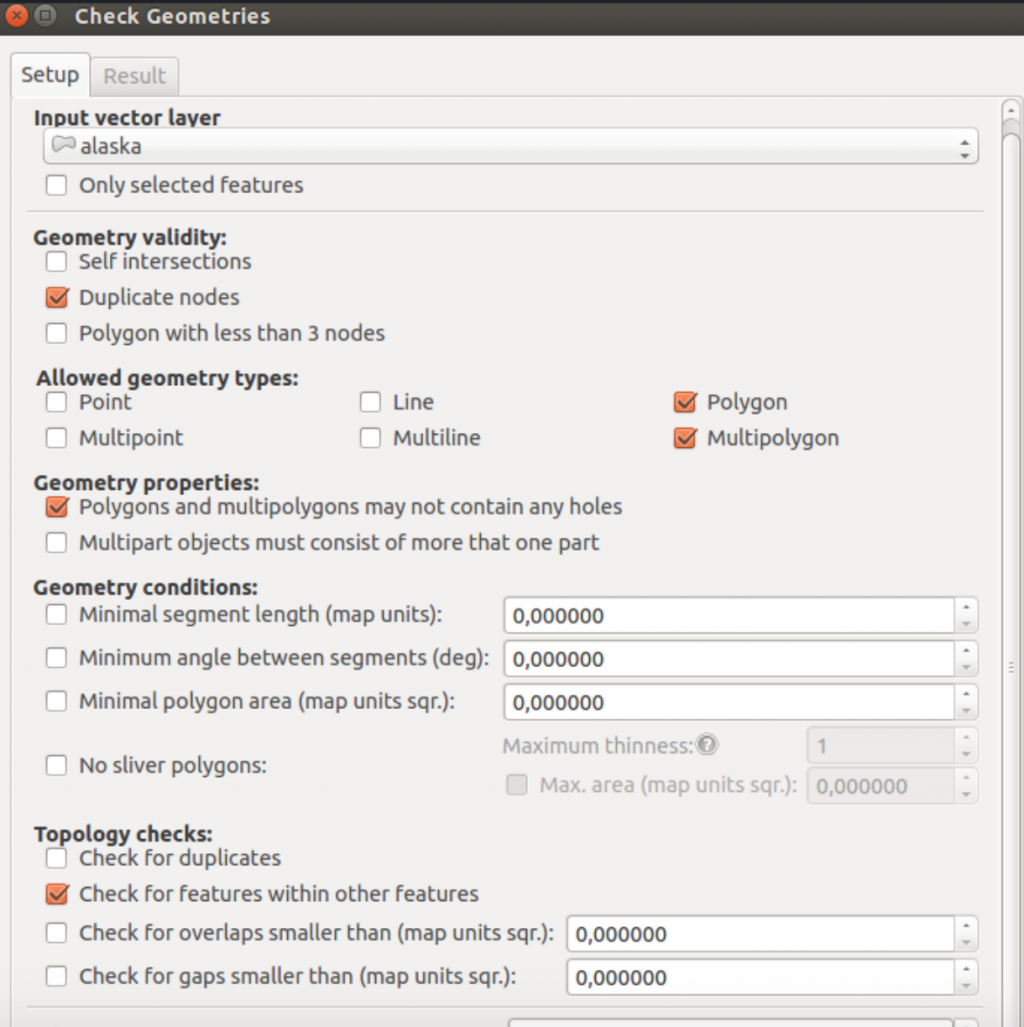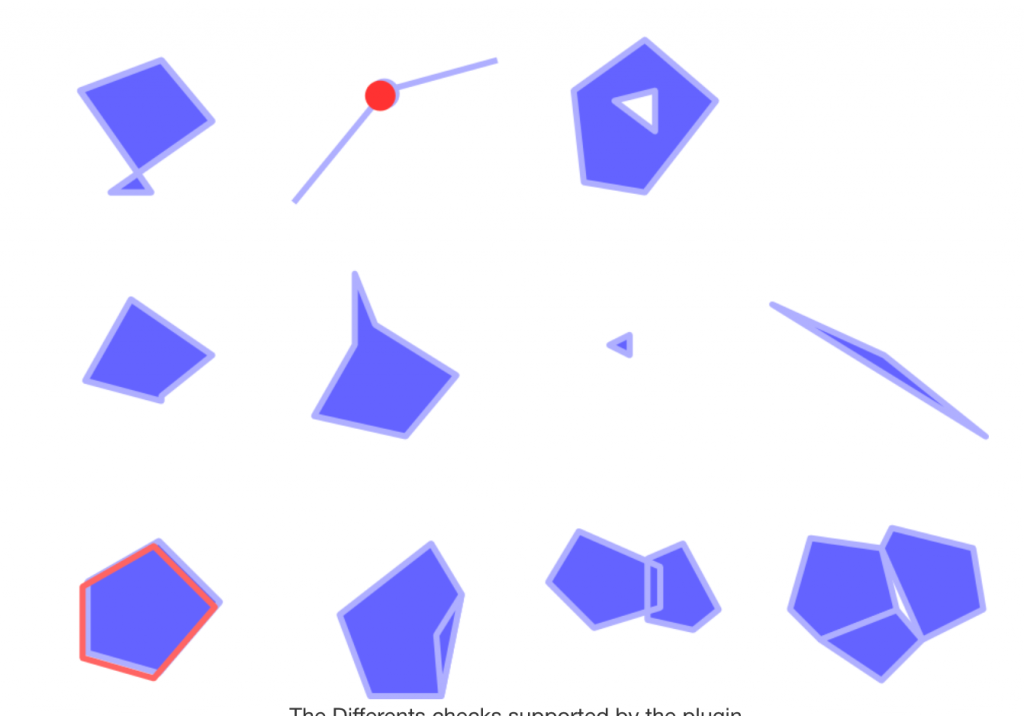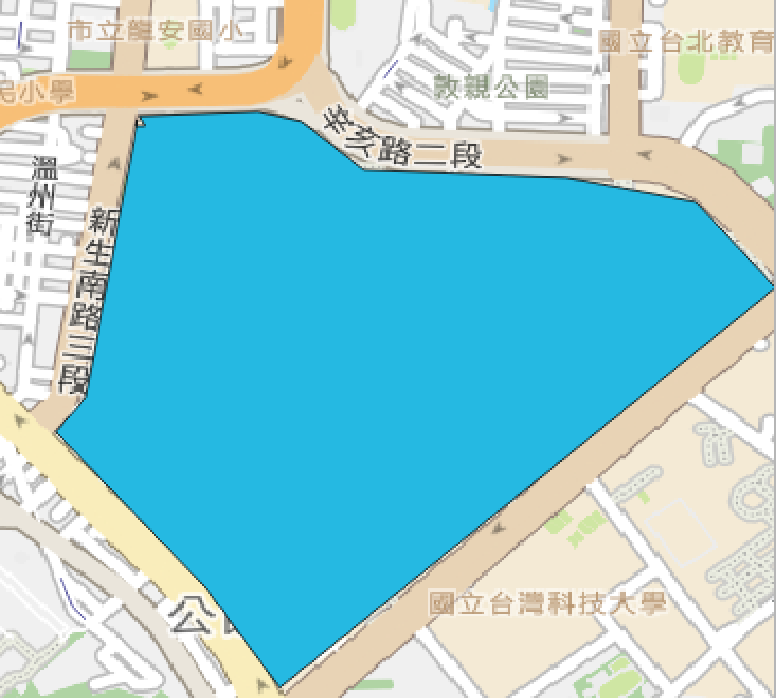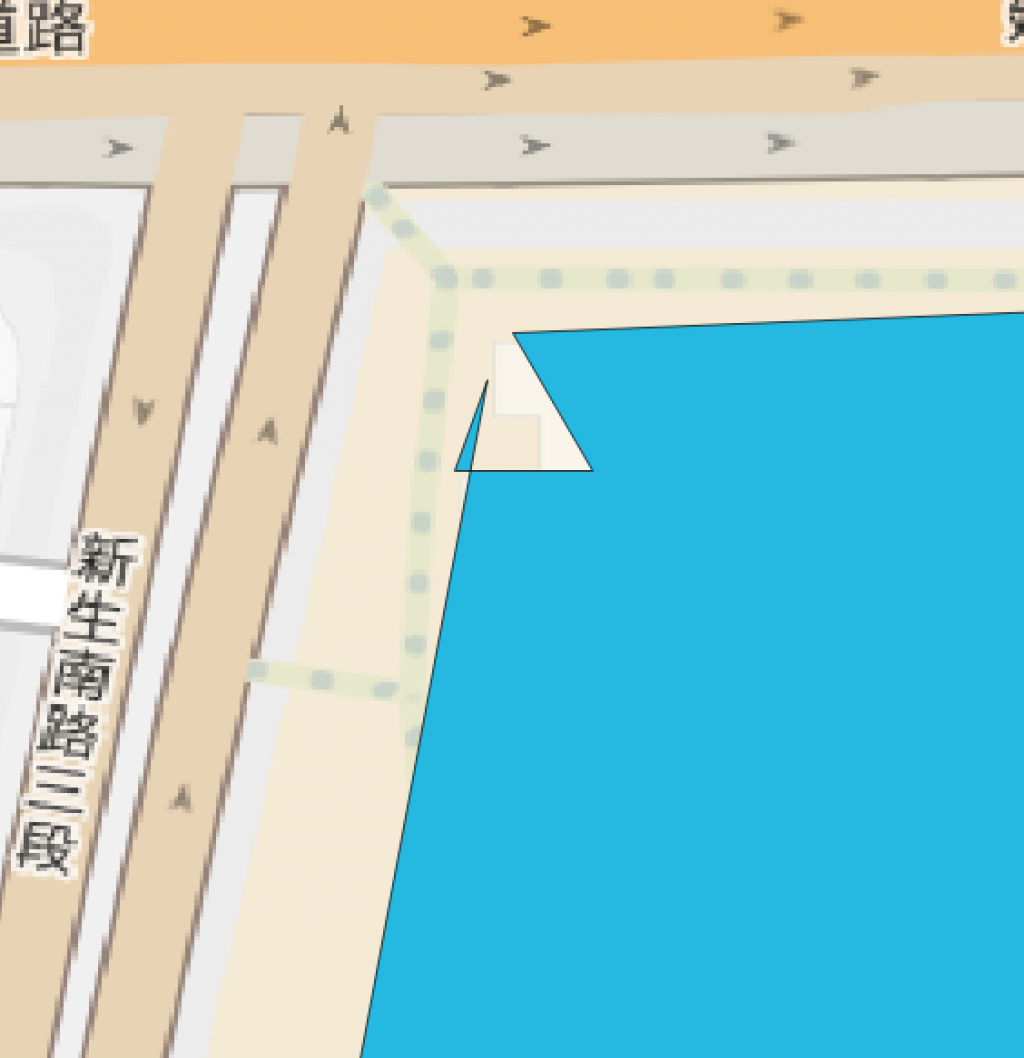#2019 iT 邦幫忙鐵人賽DAY 22
0
AI & Data

## Day22 資料品質：幾何檢查

GIS生產的過程中，在向量幾何資料部分有一些常用的位向關係檢查，這些檢查的品質指標在ArcGIS或是QGIS中都有各自的模組，例如商用軟體`ArcGIS``topology`系列模組(e.g. `Creating a topology`)。

• check geometry
• 自相交
• 重複的節點
• 多邊形小於三個節點或面積長度過小

## check geometry## 自相交

polygon的自相交應是不被允許的：

``````from shapely.geometry import Polygon
pp = Polygon([(0, 0), (1, 1), (1, -1), (0, 1)])
````````````pp.is_valid
``````

shapely: A valid Polygon may not possess any overlapping exterior or interior rings.

``````from shapely.geometry import LineString
ls=LineString(data[:]+data[0:1])
ls
````````````from shapely.ops import unary_union
mls = unary_union(ls)
mls.geom_type # MultiLineString'
``````

``````from shapely.ops import polygonize
for polygon in polygonize(mls):
print(polygon)
``````

POLYGON ((0.333 0.333, 0 0, 0 1, 0.333 0.333))
POLYGON ((0.333 0.333 0.333 0.333, 1 1, 1 -1, 0.333 0.333 0.333 0.333))

``````polygon.area
``````

(乍看問題不大)(放大來看)## 重複的節點

``````data2=[(0, 0), (1, 1),(1,1),(2,3),(0,2)]
pp2 = Polygon(data2)
pp2
````````````print(list(pp2.simplify(0).exterior.coords))
``````

## 多邊形小於三個節點或面積長度過小

``````data=[(0, 0),(0,2)]
pp = Polygon(data3)
pp ### A LinearRing must have at least 3 coordinate tuples
``````

polyhon面積不一定是檢查的項目，但可以避免錯誤

``````data=[(0, 0),(1,1),(2,3),(0,2)]
pp2= Polygon(data)
pp.area # if smaller than xxx
``````

simplify有兩種方法，preserve_topology=True僅檢查距離，如果preserve_topology=False則會使用Douglas-Peucker演算法，可以更簡化資料但是可能會有一些變形

``````data=[(0, 0),(0.002, 0.006), (1, 1),(1,1),(2,3),(0,2)]
pp = Polygon(data)
print(list(pp.simplify(0.1, ).exterior.coords))

``````

``````print(list(pp3.simplify(0.002).exterior.coords))
``````